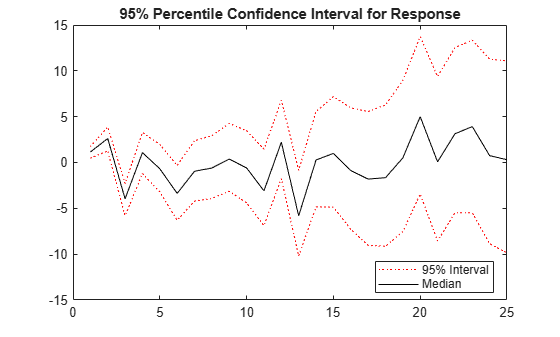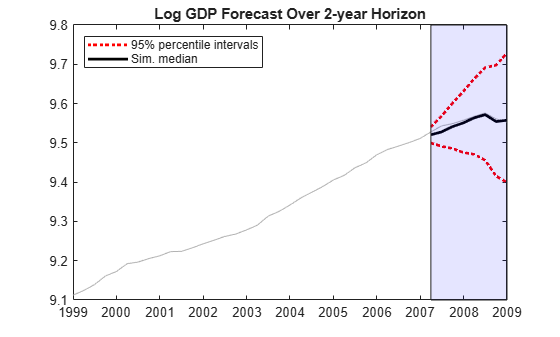# simulate

Class: regARIMA

Monte Carlo simulation of regression model with ARIMA errors

## Syntax

[Y,E] = simulate(Mdl,numObs) [Y,E,U] = simulate(Mdl,numObs) [Y,E,U] = simulate(Mdl,numObs,Name,Value) 

## Description

[Y,E] = simulate(Mdl,numObs) simulates one sample path of observations (Y) and innovations (E) from the regression model with ARIMA time series errors, Mdl. The software simulates numObs observations and innovations per sample path.

[Y,E,U] = simulate(Mdl,numObs) additionally simulates unconditional disturbances, U.

[Y,E,U] = simulate(Mdl,numObs,Name,Value) simulates sample paths with additional options specified by one or more Name,Value pair arguments.

## Input Arguments

 Mdl Regression model with ARIMA errors, specified as a regARIMA model returned by regARIMA or estimate. The properties of Mdl cannot contain NaNs. numObs Number of observations (rows) to generate for each path of Y, E, and U, specified as a positive integer.

### Name-Value Pair Arguments

Specify optional comma-separated pairs of Name,Value arguments. Name is the argument name and Value is the corresponding value. Name must appear inside quotes. You can specify several name and value pair arguments in any order as Name1,Value1,...,NameN,ValueN.

 'E0' Presample innovations that have mean 0 and provide initial values for the ARIMA error model, specified as the comma-separated pair consisting of 'E0' and a column vector or matrix. If E0 is a column vector, then it is applied to each inferred path.If E0 is a matrix, then it requires at least NumPaths columns. If E0 contains more columns than required, then simulate uses the first NumPaths columns.E0 must contain at least Mdl.Q rows. If E0 contains more rows than required, then simulate uses the latest presample innovations. The last row contains the latest presample innovation. Default: simulate sets the necessary presample innovations to 0. 'NumPaths' Number of sample paths (columns) to generate for Y, E, and U, specified as the comma-separated pair consisting of 'NumPaths' and a positive integer. Default: 1 'U0' Presample unconditional disturbances that provide initial values for the ARIMA error model, specified as the comma-separated pair consisting of 'U0' and a column vector or matrix. If U0 is a column vector, then it is applied to each inferred path.If U0 is a matrix, then it requires at least NumPaths columns. If U0 contains more columns than required, then infer uses the first NumPaths columns.U0 must contain at least Mdl.P rows. If U0 contains more rows than required, then simulate uses the latest presample unconditional disturbances. The last row contains the latest presample unconditional disturbance. Default: simulate sets the necessary presample unconditional disturbances to 0. 'X' Predictor data in the regression model, specified as the comma-separated pair consisting of 'X' and a matrix. The columns of X are separate, synchronized time series, with the last row containing the latest observations. X must have at least numObs rows. If the number of rows of X exceeds the number required, then simulate uses the latest observations. Default: simulate does not use a regression component regardless of its presence in Mdl.

### Notes

• NaNs in E0, U0, and X indicate missing values and simulate removes them. The software merges the presample data sets (E0 and U0), then uses list-wise deletion to remove any NaNs. simulate similarly removes NaNs from X. Removing NaNs in the data reduces the sample size, and can also create irregular time series.

• simulate assumes that you synchronize presample data such that the latest observation of each presample series occurs simultaneously.

• All predictors (i.e., columns in X) are associated with each response path in Y.

## Output Arguments

 Y Simulated responses, returned as a numObs-by-NumPaths matrix. E Simulated, mean 0 innovations, returned as a numObs-by-NumPaths matrix. U Simulated unconditional disturbances, returned as a numObs-by-NumPaths matrix.

## Examples

expand all

Simulate paths of responses, innovations, and unconditional disturbances from a regression model with $SARIMA\left(2,1,1{\right)}_{12}$ errors.

Specify the model:

$\begin{array}{c}{y}_{t}=X\left[\begin{array}{c}1.5\\ -2\end{array}\right]+{u}_{t}\\ \left(1-0.2L-0.1{L}^{2}\right)\left(1-L\right)\left(1-0.01{L}^{12}\right)\left(1-{L}^{12}\right){u}_{t}=\left(1+0.5L\right)\left(1+0.02{L}^{12}\right){\epsilon }_{t},\end{array}$

where ${\epsilon }_{t}$ follows a t-distribution with 15 degrees of freedom.

Distribution = struct('Name','t','DoF',15); Mdl = regARIMA('AR',{0.2, 0.1},'MA',{0.5},'SAR',0.01,... 'SARLags',12,'SMA',0.02,'SMALags',12,'D',1,... 'Seasonality',12,'Beta',[1.5; -2],'Intercept',0,... 'Variance',0.1,'Distribution',Distribution)
Mdl = regARIMA with properties: Description: "Regression with ARIMA(2,1,1) Error Model Seasonally Integrated with Seasonal AR(12) and MA(12) (t Distribution)" Distribution: Name = "t", DoF = 15 Intercept: 0 Beta: [1.5 -2] P: 27 D: 1 Q: 13 AR: {0.2 0.1} at lags [1 2] SAR: {0.01} at lag  MA: {0.5} at lag  SMA: {0.02} at lag  Seasonality: 12 Variance: 0.1 

Simulate and plot 500 paths with 25 observations each.

T = 25; rng(1) X = randn(T,2); [Y,E,U] = simulate(Mdl,T,'NumPaths',500,'X',X); figure subplot(2,1,1); plot(Y) axis tight title('{\bf Simulated Response Paths}') subplot(2,2,3); plot(E) axis tight title('{\bf Simulated Innovations Paths}') subplot(2,2,4); plot(U) axis tight title('{\bf Simulated Unconditional Disturbances Paths}')Plot the 2.5th, 50th (median), and 97.5th percentiles of the simulated response paths.

lower = prctile(Y,2.5,2); middle = median(Y,2); upper = prctile(Y,97.5,2); figure plot(1:25,lower,'r:',1:25,middle,'k',... 1:25,upper,'r:') title('\bf{95% Percentile Confidence Interval for the Response}') legend('95% Interval','Median','Location','Best')Compute statistics across the second dimension (across paths) to summarize the sample paths.

Plot a histogram of the simulated paths at time 20.

figure histogram(Y(20,:),10) title('Response Distribution at Time 20')Regress the stationary, quarterly log GDP onto the CPI using a regression model with ARMA(1,1) errors, and forecast log GDP using Monte Carlo simulation.

Load the US Macroeconomic data set and preprocess the data.

load Data_USEconModel; logGDP = log(DataTable.GDP); dlogGDP = diff(logGDP); % For stationarity dCPI = diff(DataTable.CPIAUCSL); % For stationarity numObs = length(dlogGDP); gdp = dlogGDP(1:end-15); % Estimation sample cpi = dCPI(1:end-15); T = length(gdp); % Effective sample size frstHzn = T+1:numObs; % Forecast horizon hoCPI = dCPI(frstHzn); % Holdout sample dts = dates(2:end); % Date nummbers

Fit a regression model with ARMA(1,1) errors.

Mdl = regARIMA('ARLags',1,'MALags',1); EstMdl = estimate(Mdl,gdp,'X',cpi);
 Regression with ARMA(1,1) Error Model (Gaussian Distribution): Value StandardError TStatistic PValue __________ _____________ __________ __________ Intercept 0.014793 0.0016289 9.0817 1.0685e-19 AR{1} 0.57601 0.10009 5.7548 8.6749e-09 MA{1} -0.15259 0.11978 -1.2738 0.20272 Beta(1) 0.0028972 0.0013989 2.071 0.038355 Variance 9.5734e-05 6.5562e-06 14.602 2.723e-48 

Infer unconditional disturbances.

[~,u0] = infer(EstMdl,gdp,'X',cpi);

Simulate 1000 paths with 15 observations each. Use the inferred unconditional disturbances as presample data.

rng(1); % For reproducibility gdpF = simulate(EstMdl,15,'NumPaths',1000,... 'U0',u0,'X',hoCPI);

Plot the simulation mean forecast and approximate 95% forecast intervals.

lower = prctile(gdpF,2.5,2); upper = prctile(gdpF,97.5,2); mn = mean(gdpF,2); figure plot(dts(end-65:end),dlogGDP(end-65:end),'Color',[.7,.7,.7]) datetick hold on h1 = plot(dts(frstHzn),lower,'r:','LineWidth',2); plot(dts(frstHzn),upper,'r:','LineWidth',2) h2 = plot(dts(frstHzn),mn,'k','LineWidth',2); h = gca; ph = patch([repmat(dts(frstHzn(1)),1,2) repmat(dts(frstHzn(end)),1,2)],... [h.YLim fliplr(h.YLim)],... [0 0 0 0],'b'); ph.FaceAlpha = 0.1; legend([h1 h2],{'95% Interval','Simulation Mean'},'Location','NorthWest',... 'AutoUpdate','off') axis tight title('{\bf log GDP Forecast - 15 Quarter Horizon}') hold offRegress the unit root nonstationary, quarterly log GDP onto the CPI using a regression model with ARIMA(1,1,1) errors with known intercept. Forecast log GDP using Monte Carlo simulation.

Load the US Macroeconomic data set and preprocess the data.

load Data_USEconModel; numObs = length(DataTable.GDP); logGDP = log(DataTable.GDP(1:end-15)); cpi = DataTable.CPIAUCSL(1:end-15); T = length(logGDP); frstHzn = T+1:numObs; % Forecast horizon hoCPI = DataTable.CPIAUCSL(frstHzn); % Holdout sample

Fit a regression model with ARIMA(1,1,1). The intercept is not identifiable in a model with integrated errors, so fix its value before estimation.

intercept = 5.867; Mdl = regARIMA('ARLags',1,'MALags',1,'D',1,'Intercept',intercept); EstMdl = estimate(Mdl,logGDP,'X',cpi);
 Regression with ARIMA(1,1,1) Error Model (Gaussian Distribution): Value StandardError TStatistic PValue __________ _____________ __________ ___________ Intercept 5.867 0 Inf 0 AR{1} 0.92271 0.030978 29.786 5.8706e-195 MA{1} -0.38785 0.060354 -6.4263 1.3076e-10 Beta(1) 0.0039668 0.0016498 2.4044 0.016199 Variance 0.00010894 7.272e-06 14.981 9.7343e-51 

Infer unconditional disturbances.

[~,u0] = infer(EstMdl,logGDP,'X',cpi);

Simulate 1000 paths with 15 observations each. Use the inferred unconditional disturbances as presample data.

rng(1); % For reproducibility GDPF = simulate(EstMdl,15,'NumPaths',1000,... 'U0',u0,'X',hoCPI);

Plot the simulation mean forecast and approximate 95% forecast intervals.

lower = prctile(GDPF,2.5,2); upper = prctile(GDPF,97.5,2); mn = mean(GDPF,2); figure plot(dates(end-65:end),log(DataTable.GDP(end-65:end)),'Color',... [.7,.7,.7]) datetick hold on h1 = plot(dates(frstHzn),lower,'r:','LineWidth',2); plot(dates(frstHzn),upper,'r:','LineWidth',2) h2 = plot(dates(frstHzn),mn,'k','LineWidth',2); h = gca; ph = patch([repmat(dates(frstHzn(1)),1,2) repmat(dates(frstHzn(end)),1,2)],... [h.YLim fliplr(h.YLim)],... [0 0 0 0],'b'); ph.FaceAlpha = 0.1; legend([h1 h2],{'95% Interval','Simulation Mean'},'Location','NorthWest',... 'AutoUpdate','off') axis tight title('{\bf log GDP Forecast - 15 Quarter Horizon}') hold offThe unconditional disturbances, ${u}_{t}$, are nonstationary, therefore the widths of the forecast intervals grow with time.

## References

 Box, G. E. P., G. M. Jenkins, and G. C. Reinsel. Time Series Analysis: Forecasting and Control. 3rd ed. Englewood Cliffs, NJ: Prentice Hall, 1994.

 Davidson, R., and J. G. MacKinnon. Econometric Theory and Methods. Oxford, UK: Oxford University Press, 2004.

 Enders, W. Applied Econometric Time Series. Hoboken, NJ: John Wiley & Sons, Inc., 1995.

 Hamilton, J. D. Time Series Analysis. Princeton, NJ: Princeton University Press, 1994.

 Pankratz, A. Forecasting with Dynamic Regression Models. John Wiley & Sons, Inc., 1991.

 Tsay, R. S. Analysis of Financial Time Series. 2nd ed. Hoboken, NJ: John Wiley & Sons, Inc., 2005.